# Equation + addition - math problems

#### Number of problems found: 60

• In the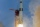In the arithmetic sequence a1 = 4.8, d = 0.4. How many consecutive members, starting with the first, need to be added so that the sum is greater than 170?
• Three integersThree consecutive integers add up to 51. what are these integers?
• Supplementary angles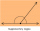(a + 30)° and (2a)° are the measure of two supplementary angles. Find the value of 'a'.
• Two numbers 11The sum of two rational numbers is (-2). If one of them is 3/5, find the other.
• Petra's testThe answer will be given 8 marks, and the wrong answer will be deducted 3 marks. The total number of marks obtained by the school is 36. If Petra answered six questions correctly,   how many questions were answered incorrectly by the student from the scho
• A piece 2A piece of material measures 38.25 inches. Courtney cuts the piece of material into two pieces. One piece measures 19.5 inches. Which addition equation could be used to find the length m of the other piece of material?
• PetraPetra likes to bathe in a bathtub with a water temperature of 38 degrees. She turned on the hot water set at 70 degrees and left for a moment. Before she returned, 40 liters of water flowed into the tub. How much water with a temperature of 22 degrees mus
• The sumThe sum of the squares of two immediately following natural numbers is 1201. Find these numbers.
• Squirrels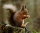The squirrels discovered a bush with hazelnuts. The first squirrel plucked one nut, the second squirrel two nuts, the third squirrel three nuts. Each new squirrel always tore one nut more than the previous squirrel. When they plucked all the nuts from the
• Angles of a hexagon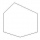Find the interior angles of a hexagon if the sizes of the angles form an arithmetic sequence, and the smallest angle is 70°.
• 1 pageOne page is torn from the book. The sum of the page numbers of all the remaining pages is 15,000. What numbers did the pages have on the page that was torn from the book?
• Finite arithmetic sequenceHow many numbers should be inserted between the numbers 1 and 25 so that all numbers create a finite arithmetic sequence and that the sum of all members of this group is 117?
• 45th birthdayThis year Mrs. Clever celebrated her 45th birthday. Her three children are now 7, 11 and 15 years old. In how many years will Mrs. Clever's age be equal to the sum of her children's years?
• Ten pupils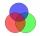10 pupils came to the art group. 8 pupils painted with watercolors and 9 pupils painted with ink, each pupil painted with ink or watercolors. How many pupils painted water and ink at the same time?
• Brother and sisterWhen I was 8, my sister was 4, and now I am 18. How old is my sister?
• Pine's forest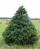There were so many pines in the forest that they were sequentially numbered 1, 2, 3,..., would use three times more digits than the pine trees alone. How many pine trees were there in the forest?55%+36%+88%+71%+100=63% what is whole (X)? Percents can be added directly together if they are taken from the same whole, which means they have the same base amount. .. . You would add the two percentages to find the total amount.
• A rectangular patioA rectangular patio measures 20 ft by 30 ft. By adding x feet to the width and x feet to the length, the area is doubled. Find the new dimensions of the patio.
• Bonuses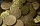Five employees of the company were paid bonuses so that each successor received 550 USD less than the previous employee. How much did everyone get if a total of USD 11,000 has paid?
• Loan 5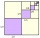Abdul takes a loan of 200000 from Ali and agrees to repay in number of instalment, each instalment begin with the 2nd exceeding the previous one by 1000, if the first instalment is 500, find how many instalment will be necessary to be wipe out the loan? C

Do you have an exciting math question or word problem that you can't solve? Ask a question or post a math problem, and we can try to solve it.

We will send a solution to your e-mail address. Solved examples are also published here. Please enter the e-mail correctly and check whether you don't have a full mailbox.

Please do not submit problems from current active competitions such as Mathematical Olympiad, correspondence seminars etc...

Do you have a linear equation or system of equations and looking for its solution? Or do you have a quadratic equation? Equations Math problems. Addition Problems.